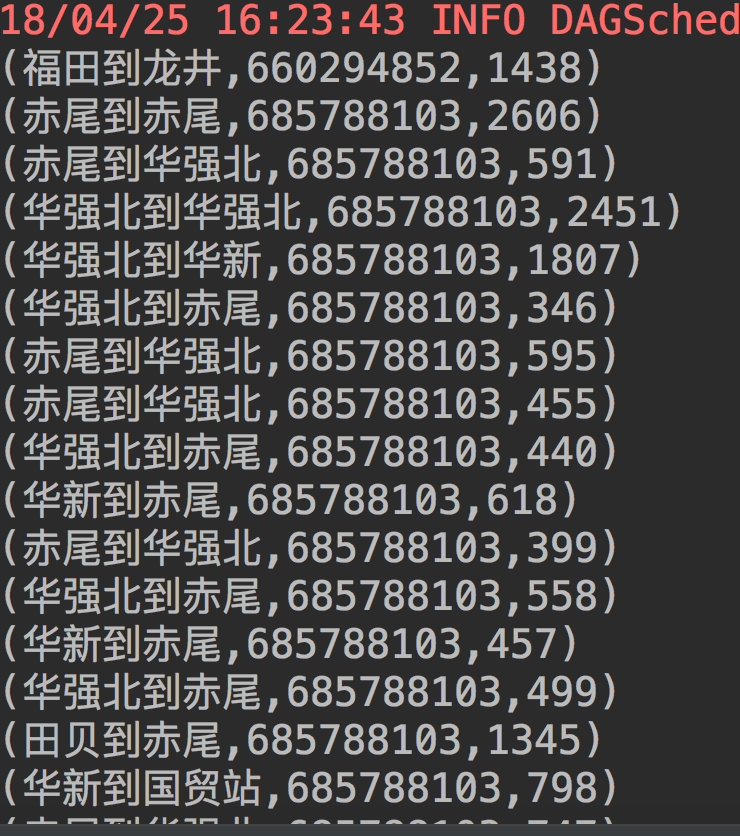2个回答

`````` from __future__ import print_function

import sys

from pyspark.sql import SparkSession

def min(a,b):
return a if a < b else b

if __name__ == "__main__":
if len(sys.argv) != 2:
print("Usage:  odcount <file>", file=sys.stderr)
exit(-1)

spark = SparkSession\
.builder\
.appName("PythonODCount")\
.getOrCreate()

lines = spark.read.text(sys.argv).rdd.map(lambda r: r)
lines = lines.filter( lambda line: len(line.strip()) > 0 )
mintimes = lines.flatMap(lambda x: [x[1:-1]]) \
.map( lambda x: (x.split(','), int(x.split(','))) ) \
.reduceByKey(min)
mintime_list = mintimes.collect()
mintime_map = {}
print("min time:")
for (od, mintime) in mintime_list:
mintime_map[od] = mintime
print("%s: %i" % (od.encode('utf-8'), mintime))

largelines = lines.flatMap(lambda x: [x[1:-1]]) \
.filter( lambda x: int(x.split(',')) > 2 * mintime_map.get(x.split(',')) )

print("large time line:")
for line in largelines.collect():
print("%s" % line.encode('utf-8'))

spark.stop()
``````qq_39582456 回复weixin_42072155: 我也知道逻辑是这样的，但是在写代码上出了问题= =weixin_42072155 好像是这样qq_39582456 可不可以麻烦你写一段代码给我看看呢，我最近才开始学Spark，很多东西都不太懂spark数据分析 求大神指教

c币c币c币

c的问题求帮助
struct subsequencern int value;rn int start;rn int end;rn;rnsubsequence maxsubsequence(int a[],int N)rnrnsubsequence maxsub;rnmaxsub.value=0;maxsub.start=0;maxsub.end=0;rnint sum=0;rnfor(int i=0;imaxsub.value)rn maxsub.value=sum;rn maxsub.end=i;rn rn else if(sum<0)rn rn sum=0;rn if(maxsub.value==0)maxsub.start=i+1;rn rn rnreturn maxsub;rnrn如果a[]=4,-5,4,5maxsub.start好象不对，if(maxsub.value==0)maxsub.start=i+1;有人能帮忙说下错在哪吗，如果这段代码没错，那么if(maxsub.value==0)maxsub.start=i+1;这又起什么作用，如果VALUE=0并不能保证最大子序一顶向下跳

spark数据分析

import java.util.*; rnrnpublic class Test rn private TreeSet ts; rn rn Test() rn this.ts = new TreeSet();rn rn rn public void addToSet(int no) rn MyClass mc = new MyClass();rn mc.a = no;rn mc.s = "";rn ts.add(mc);//当结束addToSet的调用后，mc会被当作垃圾回收吗？为什么？rn rn rn public static void main(String[] args) rn Test t = new Test();rn for (int i=0 ;i<2;i++) rn t.addToSet(i); rn rn rn rn public class MyClass rn public int a;rn public String s;rn rnrn

[code=SQL]selectrn c.付款金额,c.付款日期,c.操作人,c.操作日期,c.债务人,rn d.付款金额 as 付款金额1,rn d.付款日期 as 付款日期1,rn d.操作人 as 操作人1,rn d.操作日期 as 操作日期1,rn d.债务人 as 债务人1rnfromrn ((select a.ID as 编号,a.付款金额,a.付款日期,a.操作人,a.操作日期,b.债务人 rn from rn (select * from 欠条明细表 where 欠条编号 in (select 编号 from 欠条表 where 1=1)) a,欠条表 b rn where a.欠条编号=b.编号 and (a.id%2<>0)) c rn full outer joinrn (select a.ID as 编号,a.付款金额,a.付款日期,a.操作人,a.操作日期,b.债务人 rn from rn (select * from 欠条明细表 where 欠条编号 in (select 编号 from 欠条表 where 1=1)) a,欠条表 b rn where a.欠条编号=b.编号 and (a.id%2=0)) d on c.编号=d.编号-1)rnorder by 债务人1,c.债务人 asc[/code]rn因为里面的有删除过的数据导致的不连续....rn生成的数据表里 面里有半边是空值. 所以 想 再定义一个自动编号的字段 再排列下 然后去分单双号. 用完了就把这个字段删除掉. 但不知道怎么做?rn[url=http://topic.csdn.net/u/20081223/19/2d53fd6d-9925-4b4b-aef0-623183d01b86.html][/url]rn这是先前发的贴 .原数据都在上面

var objScript = document.createElement('script'); rnrnobjScript.id = objId; objScript.language = 'javascript'; objScript.src = url; rnrndocument.body.appendChild(objScript); rnrnrnrnrnurl = 'ceshi.ceshi.com/xao/xaodata.aspx?callback=你的js名称' rnrn请问这个动态创建脚本的代码有什么具体意义rn如果我需要从url的地址里调出一些内容进行测试,那么代码要如何写rn如何建立对远程数据的连接与显示
TO jerry_mouse() 还是昨天的问题

[url=http://bbs.csdn.net/topics/390822978]原问题[/url]rnrnrn由于昨天一些事，没有测试完全，[img=https://forum.csdn.net/PointForum/ui/scripts/csdn/Plugin/003/monkey/2.gif][/img]，又来请教大牛们了，菜鸟谢过了。rnrn[code=sql]SELECT * FROM table WHERE CHARINDEX(','+参数+',',','+列名+',')>0[/code]rn上面这句话当参数是 0,1,2,3,4,5...的时候没有问题，但是当测试的是 0_1,0_1_2....这两类数据的时候，就出现错误了。
600分求代码。。不够可以再加。

c语言问题求帮助
#include rnrnvoid main ()rnrnint x = 4, y;rny = x < 0 ? x++ : x--;rnprintf("%d %d\n" ,x, y); //3 4rnrn结果是： 3 4rn输出的y值为什么是4？怎么得来的啊？
javascript代码问题 求帮助

rn 我想在JSP页面中得到一个系统时间,然后去ORACLE数据库中比较,表中有两个字段,一个开始时间(starttime),一个结束时间(overtime),怎样在表中搜索出系统时间在两个字段之间的记录rn　这是我写的，可不对，请您指教：rnjava.util.Date t=new java.util.Date();rn String str=t.toString();rn out.print(t.toString());rn ResultSet rs=data.executeQuery("select * from zhiri where to_data('"+str+"','yyyymmdd') between starttime and overtime");
spark高级数据分析第二章

Spark高级数据分析
Spark数据分析
Spark数据分析概念入门

1.1 spark是什么 spark是一个用来实现快速而通用的集群计算的平台。 spark比mapreduce更加高效，适用于各种原先需要多种不同分布式平台的场景，包括批处理、迭代算法、交互式查询、流处理。 1.2 一个大一统的软件栈 spark的核心是一个对由很多计算任务组成的、运行在多个工作机器或者是一个计算集群上的应用进行调度、分发以及监控的计算引擎。
Spark高级数据分析.pdf
Spark高级数据分析.pdf
Spark快速数据分析-高清
《Spark快速大数据分析》高清版。机器学习大数据算法参考书 Spark作者及核心成员执笔 精通大数据处理技术。 是一本为Spark初学者准备的书，它没有过多深入实现细节，而是更多关注上层用户的具体用法。不过，本书绝不仅仅限于Spark的用法，它对Spark的核心概念和基本原理也有较为全面的介绍，让读者能够知其然且知其所以然。 　　本书介绍了开源集群计算系统Apache Spark，它可以加速数据分析的实现和运行。利用Spark，你可以用Python、Java以及Scala的简易API来快速操控大规模数据集。
spark高级数据分析

《Spark高级数据分析》

《Spark高级数据分析》源代码

C语言有不懂，求帮助
#include rnrnint main( void )rnrn char let = 'F';rn char start;rn char end;rn rn for (end = let; end >= 'A'; end--) //这里的>= 是什么意思啊？rn rn for (start = let; start >= end; start--)rn printf("%c", start);rn printf("\n");rn rn rn return 0;rnrn请帮忙解释下for循环
spark数据分析(3)
combineByKey(createCombiner,mergeValue,mergeCombiners)    createCombiner: combineByKey() 会遍历分区中的所有元素，因此每个元素的键要么还没有遇到过，要么就 和之前的某个元素的键相同。如果这是一个新的元素， combineByKey() 会使用一个叫作 createCombiner() 的函数来创建 那个键对应的...

1.Spark是什么？ Spark是一个快速而通用的集群计算平台。 快速： - spark扩展了MapReduce模型，更高效地支持更多计算模式，包括交互式查询和流处理。 - Spark能在内存中进行计算，即使是必须在磁盘上进行计算，spark仍然比MapReduce高效。 通用： - 适用于多种分布式计算场景，包括批处理、迭代算法、交互式查询、流处理。
Spark高级数据分析_完整版
Spark高级数据分析完整版